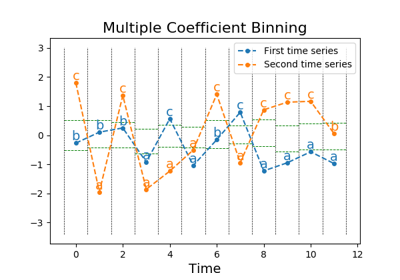# `pyts.approximation`.MultipleCoefficientBinning¶

class `pyts.approximation.``MultipleCoefficientBinning`(n_bins=4, strategy='quantile', alphabet=None)[source]

Bin continuous data into intervals column-wise.

Parameters: n_bins : int (default = 4) The number of bins to produce. It must be between 2 and `min(n_samples, 26)`. strategy : str (default = ‘quantile’) Strategy used to define the widths of the bins: ‘uniform’: All bins in each sample have identical widths ‘quantile’: All bins in each sample have the same number of points ‘normal’: Bin edges are quantiles from a standard normal distribution ‘entropy’: Bin edges are computed using information gain alphabet : None, ‘ordinal’ or array-like, shape = (n_bins,) Alphabet to use. If None, the first n_bins letters of the Latin alphabet are used if n_bins is lower than 27, otherwise the alphabet will be defined to [chr(i) for i in range(n_bins)]. If ‘ordinal’, integers are used.

References

 [Rfea62cc40411-1] P. Schäfer, and M. Högqvist, “SFA: A Symbolic Fourier Approximation and Index for Similarity Search in High Dimensional Datasets”, International Conference on Extending Database Technology, 15, 516-527 (2012).

Examples

```>>> from pyts.approximation import MultipleCoefficientBinning
>>> X = [[0, 4],
...      [2, 7],
...      [1, 6],
...      [3, 5]]
>>> transformer = MultipleCoefficientBinning(n_bins=2)
>>> print(transformer.fit_transform(X))
[['a' 'a']
['b' 'b']
['a' 'b']
['b' 'a']]
```
Attributes: bin_edges_ : array, shape = (n_bins - 1,) or (n_timestamps, n_bins - 1) Bin edges with shape = (n_bins - 1,) if `strategy='normal'` or (n_timestamps, n_bins - 1) otherwise.

Methods

 `__init__`(self[, n_bins, strategy, alphabet]) Initialize self. `fit`(self, X[, y]) Compute the bin edges for each feature. `fit_transform`(self, X[, y]) Fit to data, then transform it. `get_params`(self[, deep]) Get parameters for this estimator. `set_params`(self, \*\*params) Set the parameters of this estimator. `transform`(self, X) Bin the data.
`__init__`(self, n_bins=4, strategy='quantile', alphabet=None)[source]

Initialize self. See help(type(self)) for accurate signature.

`fit`(self, X, y=None)[source]

Compute the bin edges for each feature.

Parameters: X : array-like, shape = (n_samples, n_timestamps) Data to transform. y : None or array-like, shape = (n_samples,) Class labels for each sample. Only used if `strategy='entropy'`.
`fit_transform`(self, X, y=None, **fit_params)

Fit to data, then transform it.

Fits transformer to X and y with optional parameters fit_params and returns a transformed version of X.

Parameters: X : numpy array of shape [n_samples, n_features] Training set. y : numpy array of shape [n_samples] Target values. **fit_params : dict Additional fit parameters. X_new : numpy array of shape [n_samples, n_features_new] Transformed array.
`get_params`(self, deep=True)

Get parameters for this estimator.

Parameters: deep : bool, default=True If True, will return the parameters for this estimator and contained subobjects that are estimators. params : mapping of string to any Parameter names mapped to their values.
`set_params`(self, **params)

Set the parameters of this estimator.

The method works on simple estimators as well as on nested objects (such as pipelines). The latter have parameters of the form `<component>__<parameter>` so that it’s possible to update each component of a nested object.

Parameters: **params : dict Estimator parameters. self : object Estimator instance.
`transform`(self, X)[source]

Bin the data.

Parameters: X : array-like, shape = (n_samples, n_timestamps) Data to transform. X_new : array, shape = (n_samples, n_timestamps) Binned data.

## Examples using `pyts.approximation.MultipleCoefficientBinning`¶Multiple Coefficient Binning